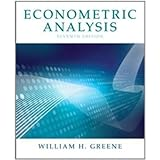# Econometric Analysis, 7th Edition### Editorial Reviews

Econometric Analysis  serves as a bridge between an introduction to the field of econometrics and the professional literature for  social scientists and other professionals in the field of social sciences, focusing on applied econometrics and theoretical background. This book provides a broad survey of the field of econometrics that allows the reader to move from here to practice in one or more specialized areas. At the same time, the reader will gain an appreciation of the common foundation of all the fields presented and use the tools they employ.

PART I: The Linear Regression Model
CHAPTER 1 Econometrics
CHAPTER 2 The Linear Regression Model
CHAPTER 3 Least Squares
CHAPTER 4 The Least Squares Estimator
CHAPTER 5 Hypothesis Tests and Model Selection
CHAPTER 6 Functional Form and Structural Change
CHAPTER 7 Nonlinear, Semiparametric, and Nonparametric Regression Models
CHAPTER 8 Endogeneity and Instrumental Variable Estimation

PART II: Generalized Regression Model and Equation Systems
CHAPTER 9 The Generalized Regression Model and Heteroscedasticity
CHAPTER 10 Systems of Equations
CHAPTER 11 Models for Panel Data

PART III: Estimation Methodology
CHAPTER 12 Estimation Frameworks in Econometrics
CHAPTER 13 Minimum Distance Estimation and the Generalized Method of Moments
CHAPTER 14 Maximum Likelihood Estimation
CHAPTER 15 Simulation-Based Estimation and Inference and Random Parameter Models
CHAPTER 16 Bayesian Estimation and Inference

PART IV: Cross Sections, Panel Data, and Microeconometrics
CHAPTER 17 Discrete Choice
CHAPTER 18 Discrete Choices and Event Counts
CHAPTER 19 Limited Dependent Variables—Truncation, Censoring, and Sample Selection
PART V: Time Series and Macroeconometrics
CHAPTER 20 Serial Correlation
CHAPTER 21 Nonstationary Data

PART VI: Appendices
Appendix A: Matrix Algebra
Appendix B: Probability and Distribution Theory
Appendix C: Estimation and Inference
Appendix D: Large-Sample Distribution Theory
Appendix E: Computation and Optimization
Appendix F: Data Sets Used in Applications

### Book Details

• Author:
• Pages: 1232 pages
• Edition: 7
• Publication Date: 2011-02-13
• Publisher:
• Language: English
• ISBN-10: 0131395386
• ISBN-13: 9780131395381

### Book Preview

Click to Look Inside This eBook: Browse Sample Pages